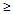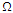# Electronic Devices - BJT Amplifiers

### Exercise :: BJT Amplifiers - General Questions

41.

Under which of the following conditions is the output impedance of the network approximately equal to RC for a common-emitter fixed-bias configuration?

 A. ro10RC B. ro < 10RC C. ro < ro D. ro > ro

Explanation:

No answer description available for this question. Let us discuss.

42.

Which of the following gains is less than 1 for a common-base configuration?

 A. Ai B. Av C. Ap D. None of the above

Explanation:

No answer description available for this question. Let us discuss.

43.

Which of the following define(s) the conversion efficiency?

 A. Ac power to the load/ac input power B. Ac power to the load/dc power supplied C. Dc output power/ac input power D. All of the above

Explanation:

No answer description available for this question. Let us discuss.

44.

The dc emitter current of a transistor is 8 mA. What is the value of re?

 A. 320B. 13.3 kC. 3.125D. 5.75Explanation:

No answer description available for this question. Let us discuss.

45.

Which of the following should be done to obtain the ac equivalent of a network?

 A. Set all dc sources to zero B. Replace all capacitors by a short-circuit equivalent. C. Remove all elements bypassed by the short-circuit equivalent. D. All of the above